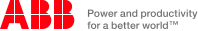# Placement Papers - ABBABB Placement Paper (Quantitative & PCM)
Posted by :
Ajantha
(31)
Paper: ABB Placement Paper (Quantitative & PCM)

1. About 10 quanti questions ( based on Mixture, Work etc. of very easy type)

2. What is GDP ?

3. Vector algebra, codition for Co-planer vector etc.

4. Gravitation, geo-synchronous satellite( it\'s hight, orbit , radius etc.), escape velocity, how g (gravitational accln) varies, about gravitational potential.

5. Basic electricity and Magnetism----Biot-savart law, current carrying conductor properties.

6. Nuclear physics, Bohr\'s constant, and Other theories related .

7. Problem based on VIBGYOR , how wave length and frequency is varying.

8. Questions based on Plank\'s Theory, E =hv

9. V=u + at , V2=u2 + 2as and W = mgh questions based on above theory

10. Faraday\'s laws of electrolysis, m = Zit

11. Heat conduction problem.

12. Co lour-coding of resistor (BBROYGBVGW)

13. How velocity of light changes in different medium while frequency remain unchanged.

14. statistics , calculation of mode, co-efficient regression (3-4 Questions)

15. f(x) = Sin x + Cos x, find the maximum value of the function.......Ans: sqrt (2)

16. Formulae for parallel plate capacitor and force between plate Comments